# 02_算法分析

### 一、算法分析

#### 1.1算法的时间复杂度分析

package main

import (
"fmt"
"time"
)

func main()  {
startTime := time.Now()
result := 0
for i := 0; i <= 100000000; i++ {
result += i
}
fmt.Println("total time:", time.Now().Sub(startTime))
}


1.算法采用的策略和方案；

2.编译产生的代码质量；

3.问题的输入规模(所谓的问题输入规模就是输入量的多少)；

4.机器执行指令的速度；

如果输入量为num为1，则需要计算1次；

func main()  {
var num, result int  // 执行一次
num = 100 // 执行一次
for i := 0; i <= num; i++ { // 执行num+1次
result += i // 执行 num 次
}

fmt.Println("result:", result)
}


如果输入量为num为1，则需要计算1次；

func main()  {
var num, result int // 执行一次
num = 100 // 执行一次
result = (num+1)*num/2 // 执行一次

fmt.Println("result:", result)
}


func sum() int {
result := 0
n := 100
for i := 1; i < n; i++ {
for j := 1; j < n; j++ {
result += j
}
}

return result
}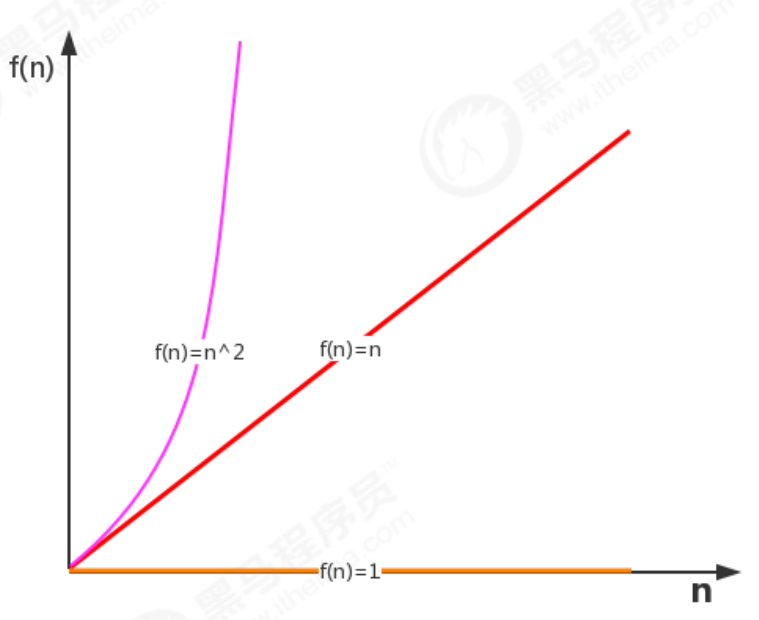##### 1.1.1 函数渐近增长

1.算法A1要做2n+3次操作，可以这么理解：先执行n次循环，执行完毕后，再有一个n次循环，最后有3次运算；

2.算法A2要做2n次操作；

3.算法B1要做3n+1次操作，可以这个理解：先执行n次循环，再执行一个n次循环，再执行一个n次循环，最后有1次运算。

4.算法B2要做3n次操作；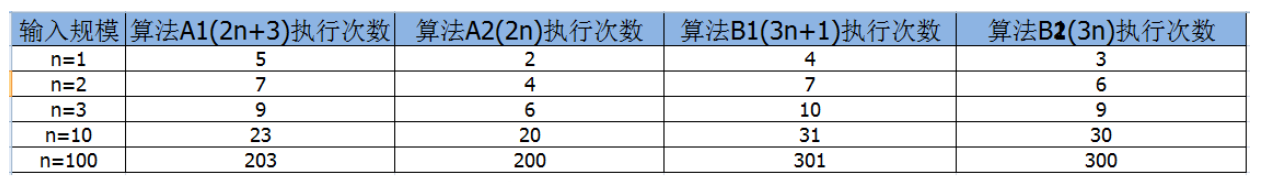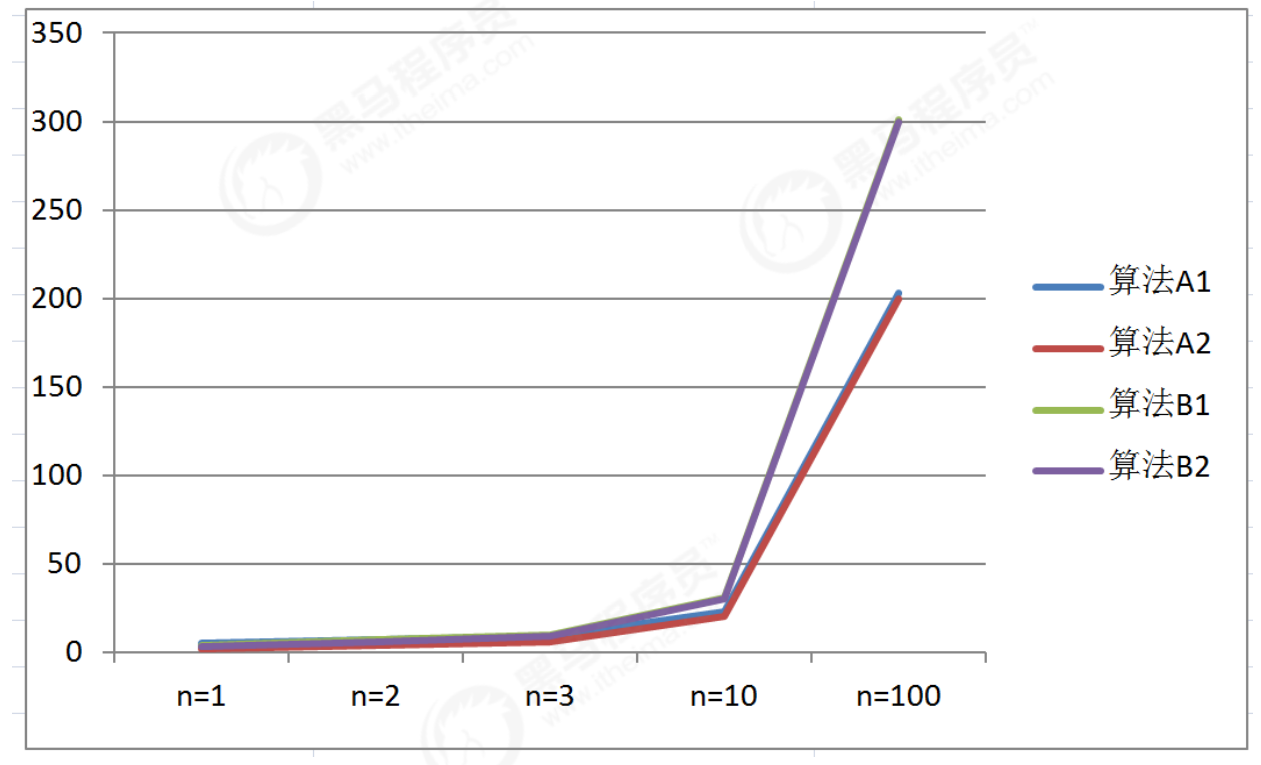1.算法C1需要做4n+8次操作

2.算法C2需要做n次操作

3.算法D1需要做2n^2次操作

4.算法D2需要做n^2次操作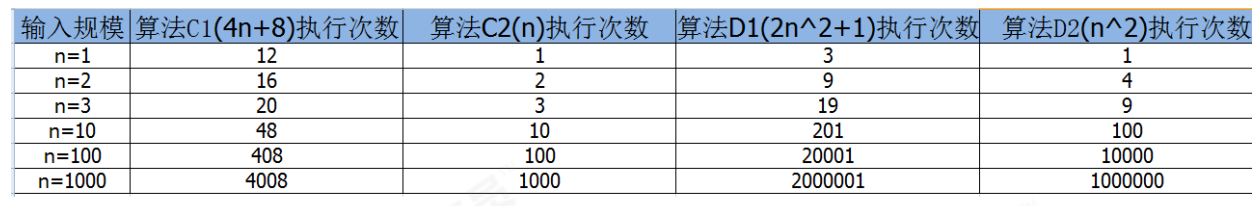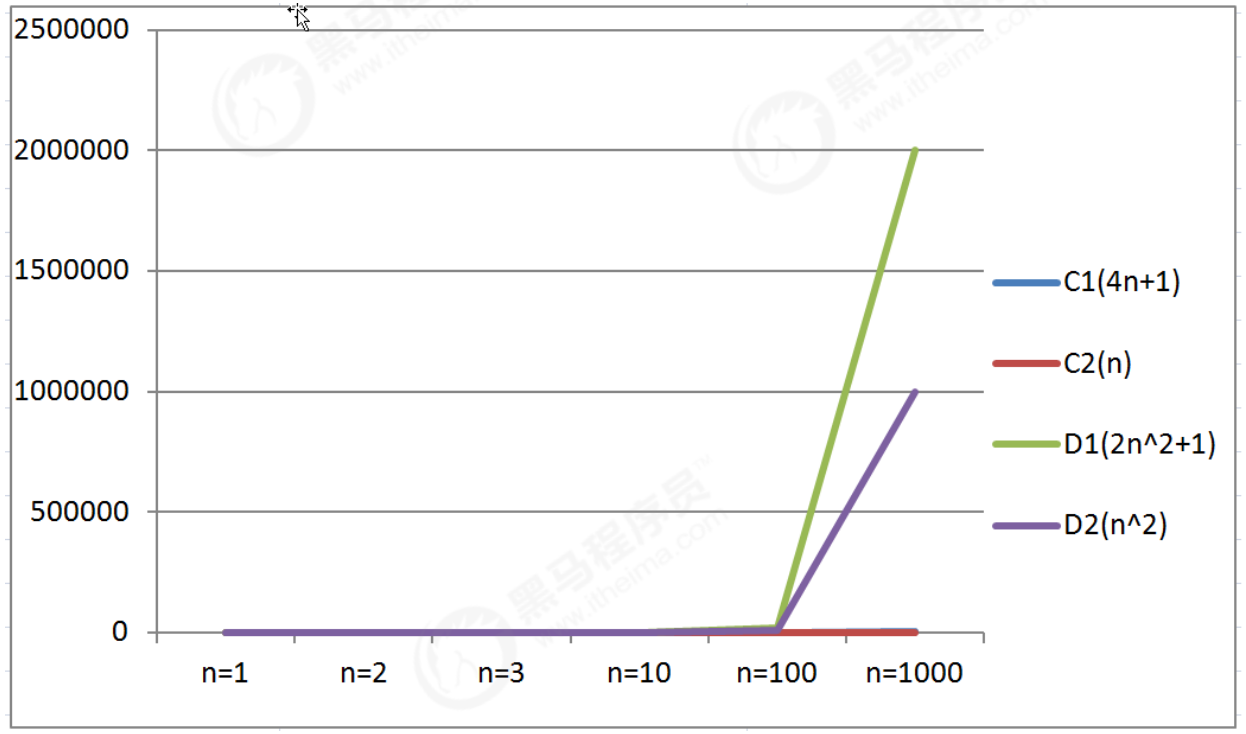2n^2+3n+1;

n^2

2n^3+3n+1

n^3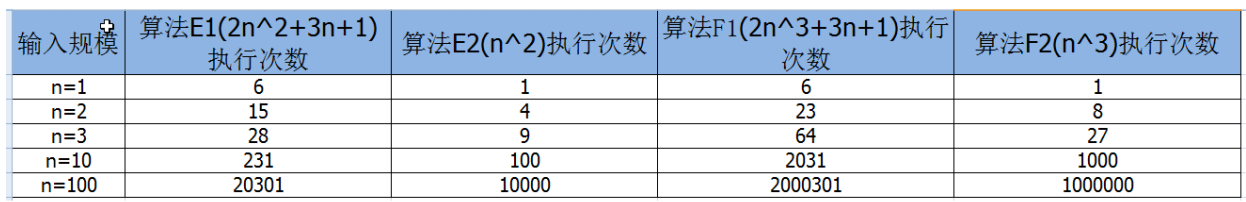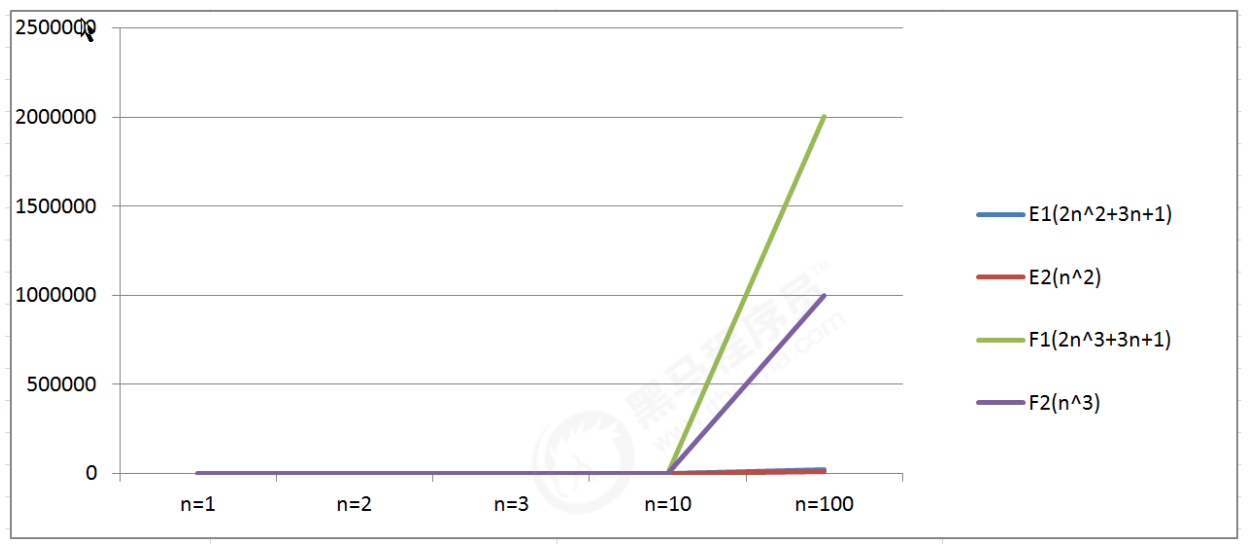n^3;

n^2;

n:

logn

1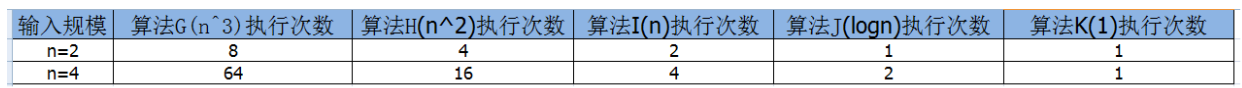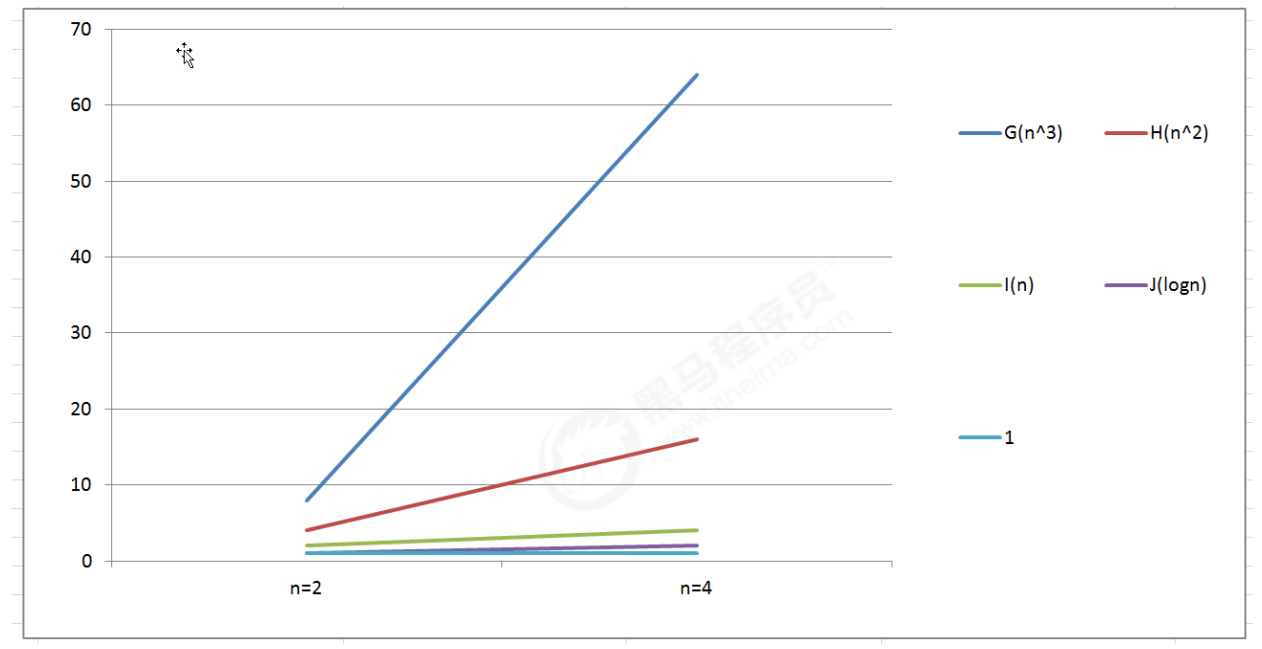1.算法函数中的常数可以忽略；

2.算法函数中最高次幂的常数因子可以忽略；

3.算法函数中最高次幂越小，算法效率越高。

##### 1.1.2算法时间复杂度
###### 1.1.2.1 大O记法

func main()  {
var num, result int // 执行一次
num = 100 // 执行一次
result = (num+1)*num/2 // 执行一次

fmt.Println("result:", result)
}


func main()  {
var num, result int  // 执行一次
num = 100 // 执行一次
for i := 0; i <= num; i++ { // 执行 n +1次
result += i // 执行 n 次
}

fmt.Println("result:", result)
}


func sum() int {
result := 0 // 执行1次
n := 100 // 执行1次
for i := 1; i < n; i++ {
for j := 1; j < n; j++ {
result += j //执行n^2次
}
}

return result
}


1.用常数1取代运行时间中的所有加法常数；

2.在修改后的运行次数中，只保留高阶项；

3.如果最高阶项存在，且常数因子不为1，则去除与这个项相乘的常数；

###### 1.1.2.2常见的大O阶

1.线性阶

func main()  {
var num, result int  // 执行一次
num = 100 // 执行一次
for i := 0; i <= num; i++ { // 执行 n +1次
result += i // 执行 n 次
}

fmt.Println("result:", result)
}


2.平方阶

func sum() int {
result := 0 // 执行1次
n := 100 // 执行1次
for i := 1; i < n; i++ {
for j := 1; j < n; j++ {
result += j //执行n^2次
}
}

return result
}


3.立方阶

func sum() int {
result := 0 // 执行1次
n := 100 // 执行1次
for i := 1; i < n; i++ {
for j := 1; j < n; j++ {
for k := 1; k < n; j++ {
result += k //执行n^3次
}
}
}

return result
}


4.对数阶

int i=1,n=100;
while(i<n){
i = i*2;
}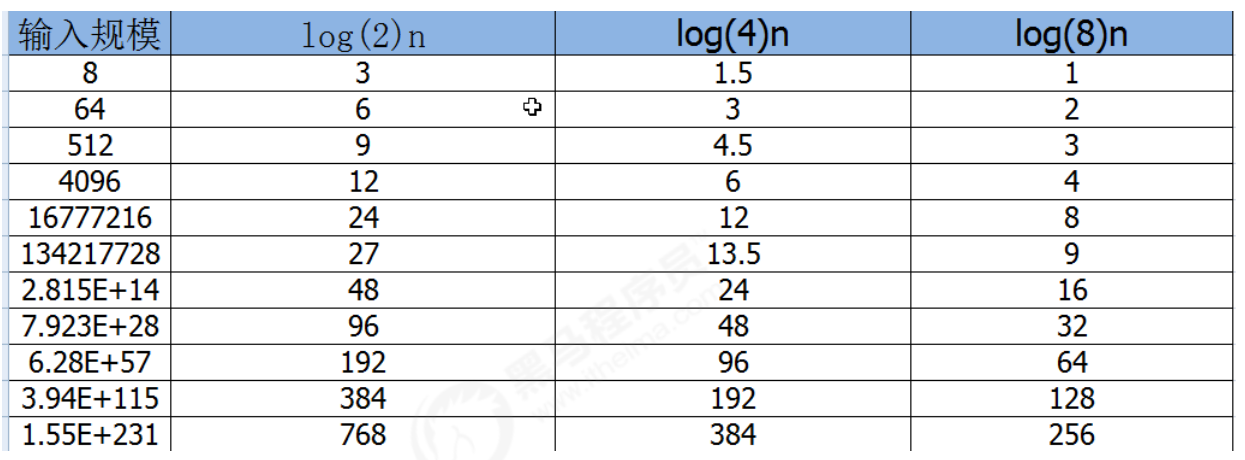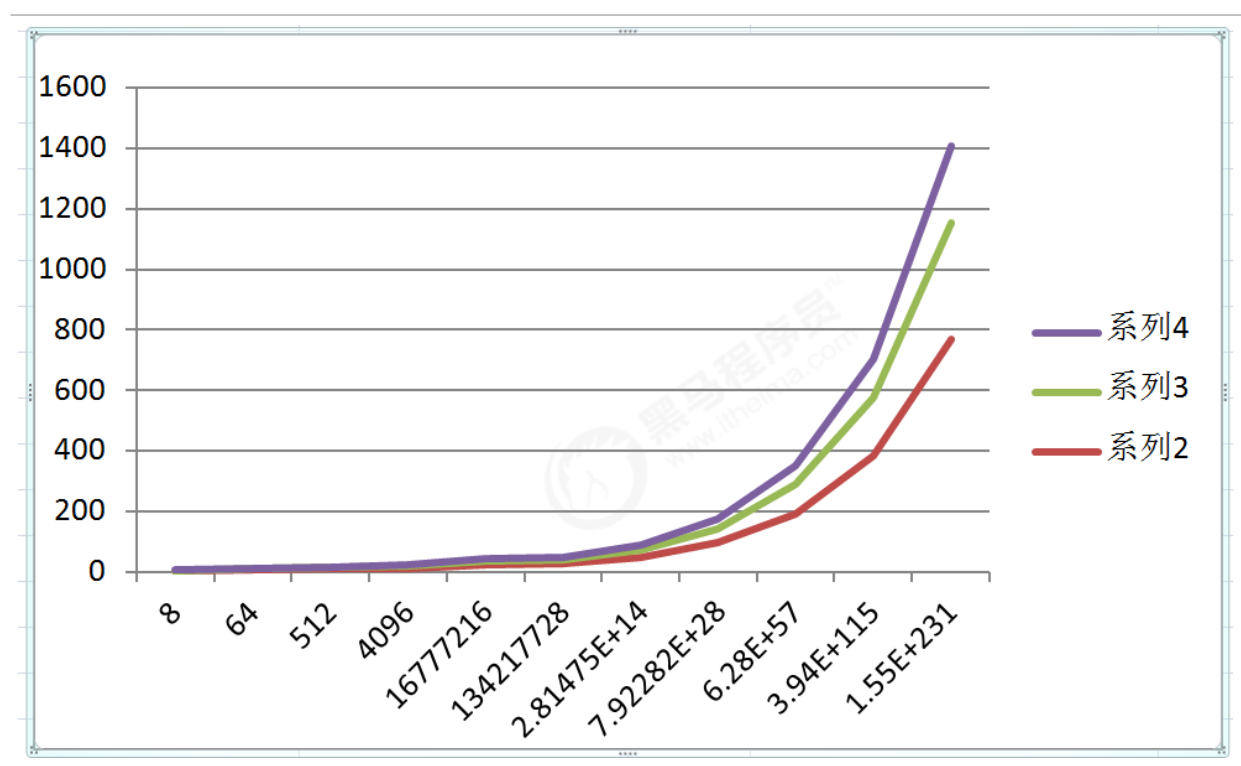5.常数阶

fun sum(n int) int {
result := n + 2
return result
}


O(1)<O(logn)<O(n)<O(nlogn)<O(n2)<O(n3)

###### 1.1.2.3 函数调用的时间复杂度分析

func main()  {
var n int  // 执行一次
n = 100 // 执行一次
for i := 0; i <= n; i++ { // 执行 n +1次
show(i) // 执行 n 次
}
}

func show(i int) {
fmt.Println("i:", i)
}


func main()  {
var n int  // 执行一次
n = 100 // 执行一次
for i := 0; i <= n; i++ { // 执行 n +1次
show(i) // 执行 n 次
}
}

func show(i int) {
for j := 0; j <= i; j++ {
fmt.Println("j:", j)
}
}


func main()  {
var n int  // 执行一次
n = 100 // 执行一次
for i := 0; i <= n; i++ { // 执行 n +1次
show(i) // 执行 n 次
}

for i := 1; i < n; i++ {
for j := 1; j < n; j++ {
fmt.Println(j)
}
}

}

func show(i int) {
for j := 0; j <= i; j++ {
fmt.Println("j:", j)
}
}


###### 1.1.2.4最坏情况

func search(num int) int {
result := []int{11, 22, 4, 6, 54, 89}
for i := 0; i < len(result); i++ {
if num == i {
return i
}
}
return -1
}


##### 1.2.1 golang 中常见内存占用

1.基本数据类型内存占用情况：

2.计算机访问内存的方式都是一次一个字节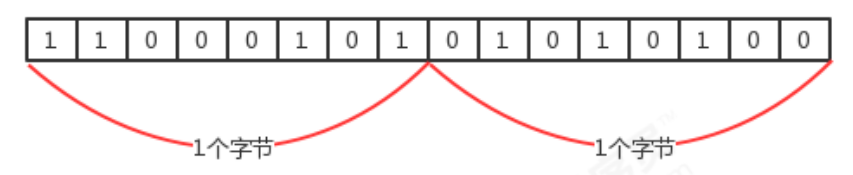1.2.2 算法的空间复杂度

package main

import (
"fmt"
)

func main()  {
a := []int{1,2,5, 6}
fmt.Println(reverse(a))
}

func reverse(arr []int) []int {
n := len(arr) // 8字节
var tmp int // 8字节

end := n - 1 // 8字节
for start := 0; start <= end; start++ {
tmp = arr[start]
arr[start] = arr[end]
arr[end] = tmp
end--
}

return arr
}


func reverse2(arr []int) []int {
n := len(arr) // 8字节
tmp := make([]int, n) // 8*n 字节

for i := n - 1; i >= 0; i-- {
tmp[n-1-i] = arr[i]
}
return tmp
}


8+8n+数据头部信息占用的字节

posted @ 2023-01-15 17:12  画个一样的我  阅读(44)  评论(0编辑  收藏  举报